# Expansion

If one side of the rectangle is larger 4-times and second 2-times, what percentage increases the area of rectangle?

p =  700 %

### Step-by-step explanation:

$p=100\cdot \left(4\cdot 2-1\right)=700\mathrm{%}$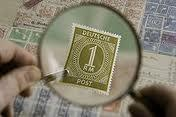Did you find an error or inaccuracy? Feel free to write us. Thank you!Tips to related online calculators
Check out our ratio calculator.

#### You need to know the following knowledge to solve this word math problem:

We encourage you to watch this tutorial video on this math problem:

## Related math problems and questions:

• Ratio - rectangleThe rectangle has dimensions 6 cm and 9 cm. How many times increases area and its perimeter, when its dimensions increase in the ratio 5:3?
• Percentage and rectangleAbout what percentage increases perimeter and area of a rectangle if both the sides 12 cm and 10 cm long we increase by 20%?
• Ratio of sides 2The ratio of the side lengths of one square to another is 1:2. Find the ratio of the area of the two squares.
• In theIn the national park, the ratio of the wooded area to grassland is 4: 1. The total area is 385km2. What area is wooded?
• Negative percentage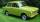In 2006, the company had a loss of 11100 Euros. Two years later, he was already in profit 50000 Eur. Calculate what percentage of the company increased profits in these two years.
• Rectangle 3-4-5The sides of the rectangle are in a ratio of 3:4. The length of the rectangle diagonal is 20 cm. Calculate the content of the rectangle.
• ScaleThe swimming pool is long 110 m and 30 m wide. The plan of the city is shown as a rectangle with an area 8.25 cm2. What scale is the city plan?
• DivideDivide area of rectangles with dimensions 32m and 10m by the ratio 7: 9. What area corresponds to a smaller section?
• Sides of a rectangleThe dimensions of a rectangle are in a 4:12 ratio. If the shorter side length is 12 cm, what is the length of the longer side in centimetres?
• Square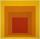If we increase side of the square, increase its area of 63%. What is the percentage we increase side of the square?
• Rectangles - sidesOne side of the rectangle is 10 cm longer than a second. Shortens longer side by 6 cm and extends shorter by 14 cm increases the rectangle area by 130 cm2. What are the dimensions of the original rectangle?
• Square to rectangle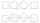What is the ratio of the area of a square of side x to the area of a rectangle of a rectangle of width 2 x and length 3
• The sidesThe sides of the rectangle are in a ratio of 3: 5 and its circumference measures 72 cm. Calculate: a) the size of both sides of the rectangle b) the area of the rectangle c) the length of the diagonals
• Percentage of waste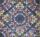In a square plate with side 75 cm we cut 4 same circles. Calculate the percentage of waste.
• Olda wanted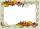Olda wanted to enlarge the 9 cm x 13 cm photo so that the shorter side measured 18 cm. How big will the longer side of the photo be?
• GardenThe rectangular garden has dimensions of 27 m and 30 m. Peter and Katka split it in a ratio of 4:5. How many square meters did Katkin measure part of the garden?
• CZ flag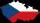What percentage of the Czech flag is made up of blue, white and red textiles?